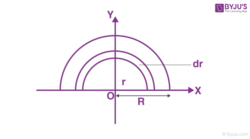Win up to 100% scholarship on Aakash BYJU'S JEE/NEET courses with ABNAT Win up to 100% scholarship on Aakash BYJU'S JEE/NEET courses with ABNAT

# Centre of Mass of Semicircular Disc

Centre of Mass is a fixed point on the object about which the entire mass of the system is equally distributed. Knowing the value of the centre of mass is useful in solving mechanics problems, where we have to describe the motion of unevenly shaped objects and complicated systems. For calculation purposes, we can consider that the mass of the entire oddly shaped objects is concentrated in a tiny object kept at the centre of mass. In this article, we will discuss the steps to find the centre of mass of the semicircular disc.

## How to Find the Centre of Mass of Semicircular DiscLet M be the mass of the semicircular disc of radius R, then the density or mass per unit area of the disc is

σ = M/(πR2/2) = 2M/πR2

Area of the element is = ½[π(r+dr)2 – πr2]

= πrdr (∵(dr)2 ≪0)

Elementary ring mass, dM = (πrdr)σ = (πrdr)(2M/πR2) = (2Mr/R2)dr

If (x,y) is the coordinates of the centre of mass of this element,

Then (x,y) = (0,2r/π)

Therefore x = 0 and y = 2r/π

Let xcm and ycm be the coordinates of the centre of mass of the semicircular disc.

Then

xcm= 0 (due to symmetry)

ycm = (1/M)∫ydM

Substitute the value of y and dM

$$\begin{array}{l}y_{cm} = \frac{1}{M}\int_{0}^{R}\left ( \frac{2r}{\pi } \right ) \frac{2Mr}{R^{2}}dr\end{array}$$

$$\begin{array}{l}y_{cm} = \frac{1}{M}\int_{0}^{R}\left ( \frac{2r}{\pi } \right ) \frac{2Mr}{R^{2}}dr\end{array}$$

yCM = (4/πR2)(R3/3) = 4R/3π

## Recommended Videos

### Centre of Mass and Collisions – Video Lesson### Centre of Mass and Collision – Jee Physics Important TopicsTest Your Knowledge On Centre Of Mass Of Semicircular Disc!